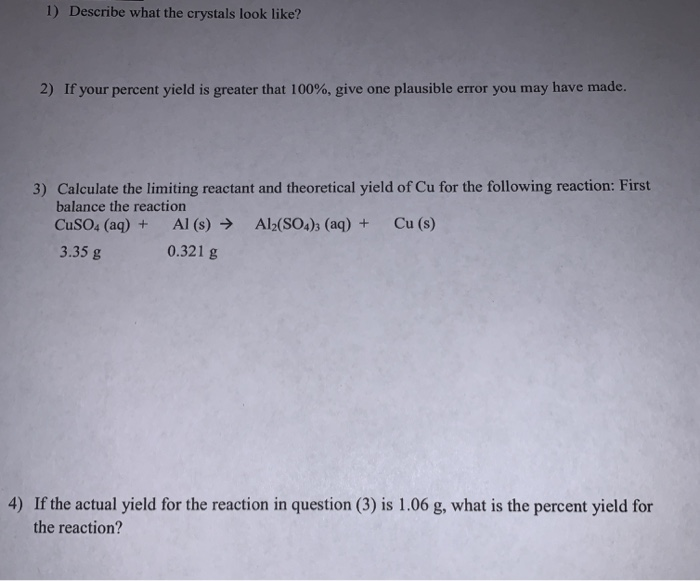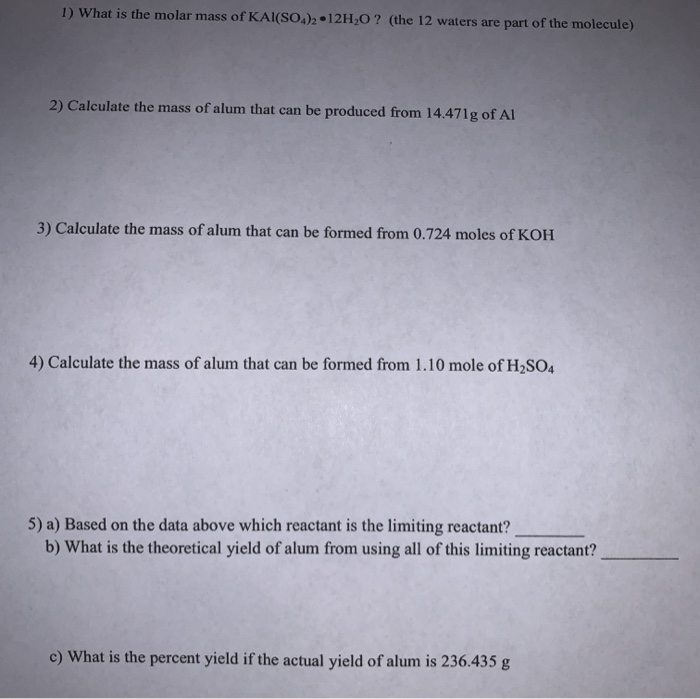# Help with all questions(: 1) Describe what the crystals look like? 2) If your percent yield...

###### Question:

help with all questions(:1) Describe what the crystals look like? 2) If your percent yield is greater that 100%, give one plausible error you may have made. 3) Calculate the limiting reactant and theoretical yield of Cu for the following reaction: First balance the reaction CuSO4 (aq) + Al(s) → Al2(SO4)(aq) + Cu (S) 3.35 g 0.321 g 4) If the actual yield for the reaction in question (3) is 1.06 g, what is the percent yield for the reaction?
1) What is the molar mass of KAl(SO )2 • 12H,0? (the 12 waters are part of the molecule) 2) Calculate the mass of alum that can be produced from 14.471g of Al 3) Calculate the mass of alum that can be formed from 0.724 moles of KOH 4) Calculate the mass of alum that can be formed from 1.10 mole of H2SO4 5) a) Based on the data above which reactant is the limiting reactant? b) What is the theoretical yield of alum from using all of this limiting reactant? c) What is the percent yield if the actual yield of alum is 236.435 g

#### Similar Solved Questions

##### The reform reaction between steam and gaseous methane (CH) produces "synthesis gas," a mixture of carbon...
The reform reaction between steam and gaseous methane (CH) produces "synthesis gas," a mixture of carbon monoxide gas and dihydrogen gas. Synthesis gas is one of the most widely used industrial chemicals, and is the major industrial source of hydrogen Suppose a chemical engineer studying a n...